Courses

# Test: Single Phase HW AC-DC - 2

## 20 Questions MCQ Test Power Electronics | Test: Single Phase HW AC-DC - 2

Description
This mock test of Test: Single Phase HW AC-DC - 2 for Electrical Engineering (EE) helps you for every Electrical Engineering (EE) entrance exam. This contains 20 Multiple Choice Questions for Electrical Engineering (EE) Test: Single Phase HW AC-DC - 2 (mcq) to study with solutions a complete question bank. The solved questions answers in this Test: Single Phase HW AC-DC - 2 quiz give you a good mix of easy questions and tough questions. Electrical Engineering (EE) students definitely take this Test: Single Phase HW AC-DC - 2 exercise for a better result in the exam. You can find other Test: Single Phase HW AC-DC - 2 extra questions, long questions & short questions for Electrical Engineering (EE) on EduRev as well by searching above.
QUESTION: 1

### Choose the correct statement with respect to the below given circuit.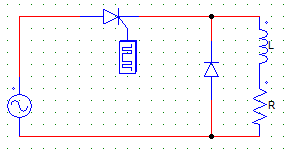Solution:

The voltage cannot be negative due to the FD (freewheeling diode or commutating diode so connected).

QUESTION: 2

### In the below given circuit, the FD (Freewheeling diode) is forward biased at ωt =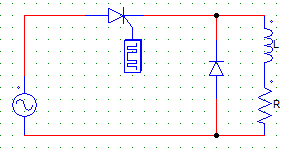Solution:

It is forward biased at π by the conducting SCR & the current starts to through the FD & Load.

QUESTION: 3

### In the below given circuit, when the commutating diode or FD is conducting than the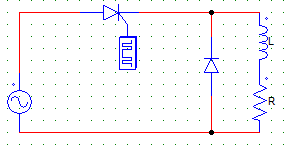Solution:

When the FD is forward biased at π by the conducting SCR & the current starts to through the FD & load.

QUESTION: 4

The output voltage waveform of the below given circuit would be the same that obtained from a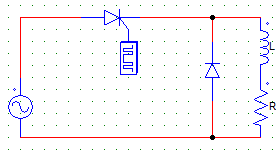Solution:

The wave from will be like a half wave diode rectifier circuit. Which is the same as that obtained from a half-wave R load circuit and the above given circuit.

QUESTION: 5

In a single-phase half-wave circuit with RL load and a freewheeling diode, the load voltage during the freewheeling period will be

Solution:

The FD short circuits the load and voltage across a short circuit would be = 0.

QUESTION: 6

In a single-phase half-wave circuit with RL load and a freewheeling diode, the freewheeling period is

Solution:

Freewheeling period is the one in which the FD diode conducts.

QUESTION: 7

A single-phase half wave rectifier with a FD is supplied by Vs = 240 V, AC with a load R = 10 Ω, L = 0.5 mH and a firing angle α = 30°. Find the average value of the load voltage.

Solution:

Vo = (Vm/2π) x (1+cosα)
Vm = √2Vs.

QUESTION: 8

A single-phase HW rectifier with a FD is supplied by Vs = 240 V, 50 Hz, AC with a load R = 10 Ω, L = 0.5 mH and a firing angle α = 30°. Find the average value of the load current.

Solution:

Vo = (Vm/2π) x (1+cosα)
Vm = √2Vs
Io = Vm/R (Due to the FD).

QUESTION: 9

A single phase half-wave controlled rectifier has 400 sin314t as the input voltage and R as the load. For a firing angle of 60°,the average output voltage is

Solution:

Vo = (Vm/2π) x (1+cosα) = 400/2π x (1+cos60) = 300/π.

QUESTION: 10

Choose the incorrect statement with respect to the use of FD in half-wave circuits.

Solution:

PIV is unaffected with the use of FD (freewheeling diodes).

QUESTION: 11

A single-phase half-wave rectifier with a FD is supplied by Vs = 240 V, 50 Hz, AC source with a load R = 10 Ω, L = 0.8 mH. The firing angle is so adjusted such that the output voltage obtained is 100 V. Find the firing angle.

Solution:

Vo = Vm/2π cos(firing angle).

QUESTION: 12

For the below shown circuit, a motor load (RLE) is connected to the SCR through supply Vs. The minimum value of the firing angle to turn on the SCR would be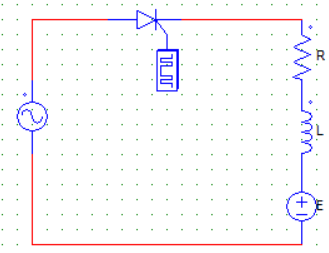Solution:

The firing angle should be such that the value of V exceeds E. So SCR turns on at excatlly Vm sinωt = E
Therefore ωt = min. angle = Sin-1(E/Vm).

QUESTION: 13

Choose the correct statement with respect to the below given circuit.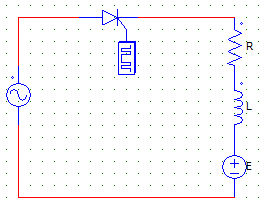Solution:

Even if no current is flowing through the load when the SCR is off, voltage = E will exists at the load terminals at all times.

QUESTION: 14

By using a freewheeling diode (FD) in a rectifier with RL load, the power consumed by the load

Solution:

The FD feeds inductor current again to the load.

QUESTION: 15

A 230 V, 50 Hz, one-pulse SCR controlled converter has extinction angle β = 210°. Find the circuit turn-off time

Solution:

t = 2π-β/ω
Where, π = 90°
ω = 2xπx50
β = 210°.

QUESTION: 16

A 230V, 50Hz, single-pulse SCR is feeding a RL load with α = 40° and β = 210°. Find the value of average output voltage

Solution:

Vo = Vm/2π x (cosα-cosβ)
Where Vm = √2Vs.

QUESTION: 17

A single-pulse transformer with secondary voltage of 230 V, 50 Hz, delivers power to bulb of R = 10 Ω through a half-wave controlled rectifier circuit. For α = 60°, find the average current in the bulb

Solution:

First find the average voltage, than Io = Vo/R
Vo = (Vm/2π) x (1+cosα).

QUESTION: 18

A single-pulse transformer with secondary voltage of 230 V, 50 Hz, delivers power to bulb of R = 10 Ω through a half-wave controlled rectifier circuit. For α = 60° and output AC power of 2127 Watts, find the rectification efficiency

Solution:

Vo = (Vm/2π) x (1+cosα) = 77.64 V
Pdc = Vo2xR = 602.8 W
Rectification efficiency = Pdc/Pac = 28.32 %.

QUESTION: 19

Find the PIV of the SCR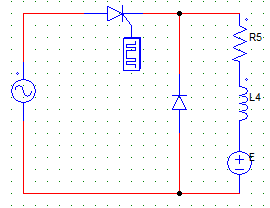Solution:

The maximum negative voltage faced by the SCR is –Vm which is nothing but √2Vs.

QUESTION: 20

An SCR rectifier circuit is designed such that the average output voltage is 77.64 V & RMS value of output voltage is 145.873 V. Find the voltage ripple factor.

Solution:

FF = 145.873/77.64 = 1.879
VRF = √(FF2-1) = 1.5908.